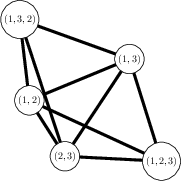# How to draw a graph whose vertices are elements of permutation groupHow to write the following program in SageMath:

Consider the Permutation Group $S_3$.

The elements of $S_3$ are $e,(12),(13),(23),(123),(132)$.

I want to draw a graph $G$ whose members are the elements of $S_3$ and two vertices $x,y$ are adjacent if and only if $xy\neq yx$.

I am stuck in doing the following things:

1. How to call the elements of $S_3$ through a loop?
2. How to draw the graph $G$ ?

I can check whether they commute or not but I am stuck in the two things. Is there any way to write the code in SageMath?

As an example if I input $S_3$ I want to get the following graph

$G=Graph({1:[2,3,4,5],2:[1,3,4,5],3:[1,2,4,5],4:[1,2,3],5:[1,2,3]})$

Any help will be highly appreciated.

edit retag close merge delete

Sort by » oldest newest most votedFor #1:

sage: G = groups.permutation.Symmetric(3)
sage: for x in G:
....:       (do stuff with x)


For example:

sage: G = groups.permutation.Symmetric(3)
sage: d = {}
sage: for x in G:
....:     for y in G:
....:         if x*y != y*x:
....:             if x in d:
....:                 d[x].append(y)
....:             else:
....:                 d[x] = [y]
....:


At this point, d is a dictionary. The keys are the non-identity elements of G, and the value corresponding to x is the list of elements y that do not commute with x. So for #2:

sage: gr = Graph(d)
sage: view(gr)more

I am extremely thankful for the answer sir. Can you kindly explain the logic behind "if x in d: d[x].append(y) else d[x]=[y]" If you could explain why is it written this way I will be grateful

d[x] is supposed to be a list consisting of the elements y which do not commute with x. If that list hasn't been created yet (because no such elements y have been found), then if x in d will return False, so you set d[x] to be the list containing y. Otherwise d[x] exists, so append y to the end of the list.

Note that using a defaultdict(list) (imported from collections) instead of a dict, you could replace those 4 lines with d[x].append(y)